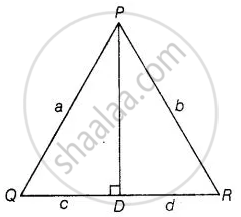# In ∆PQR, PD ⊥ QR such that D lies on QR. If PQ = a, PR = b, QD = c and DR = d, prove that (a + b)(a – b) = (c + d)(c – d). - Mathematics

Sum

In ∆PQR, PD ⊥ QR such that D lies on QR. If PQ = a, PR = b, QD = c and DR = d, prove that (a + b)(a – b) = (c + d)(c – d).

#### Solution

Given In ∆PQR, PD 1 QR, PQ = a, PR = b,QD = c and DR =d

To prove (a + b) (a-b) = (c + d)(c-d)

Proof In right angled ΔPDQ,

PQ^2 = PD^2 + QD^2   .....[By Pythagoras theorem]

⇒ a^2 = PD^2 + c^2

⇒ PD^2 = a^2 - c^2  .....(i)In right-angled ∆PDR,

PR^2 = PD^2 + DR^2   .......[By Pythagoras theorem]

⇒ b^2 = PD^2 + d^2

⇒ PD^2 = b^2 - d^2  .....(ii)

From equations (i) and (iii),

a^2 - c^2 = b^2 - d^2

⇒ a^2 - b^2 = c^2 - d^2

⇒ (a - b)(a + b) = (c - d)(c + d)

Hence proved

Concept: Basic Proportionality Theorem (Thales Theorem)
Is there an error in this question or solution?

#### APPEARS IN

NCERT Mathematics Exemplar Class 10
Chapter 6 Triangles
Exercise 6.4 | Q 11 | Page 74

Share